# Chemical Equations and Reactions Describing Chemical Reactions Indications

• Slides: 27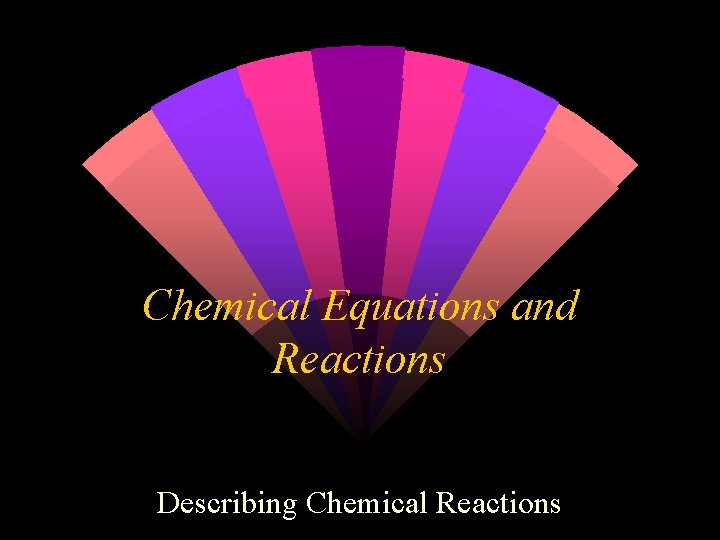Chemical Equations and Reactions Describing Chemical ReactionsIndications of a chemical reaction A chemical reaction is the process by which one or more substances are changed into one or more different substances. w A chemical equation represents with symbols and formulas (or with words), the identities and the relative amounts of the reactants and products. w The original substances are called reactants and are shown on the left side of the equation. w The substances formed are called products and are shown on the right side of the equation. wChemical symbols seen in a chemical equation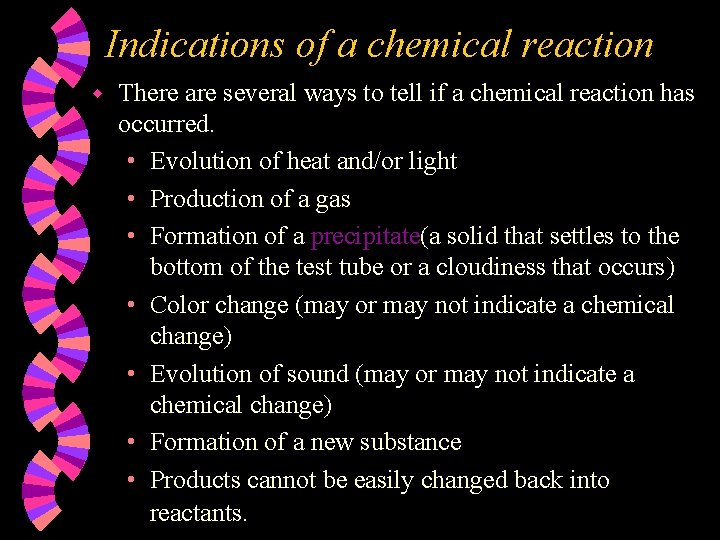Indications of a chemical reaction w There are several ways to tell if a chemical reaction has occurred. • Evolution of heat and/or light • Production of a gas • Formation of a precipitate(a solid that settles to the bottom of the test tube or a cloudiness that occurs) • Color change (may or may not indicate a chemical change) • Evolution of sound (may or may not indicate a chemical change) • Formation of a new substance • Products cannot be easily changed back into reactants.Characteristics of a chemical equation The equation must represent the facts. All reactants and products must be identified by chemical analysis. w The equation must contain the correct formulas for all substances involved. w • Remember to use oxidation states when writing formulas. • Remember that some elements are diatomic and must have a 2 as a subscript when written in an equation.Diatomic elementsCharacteristics of a chemical equation w The law of conservation of mass must be observed at all times. Atoms may not be created or destroyed but may be rearranged to make new substances. • To equalize the number of atoms on both sides of an equation, coefficients are used. It is placed in front of the compound. • NEVER change the subscripts in a formula.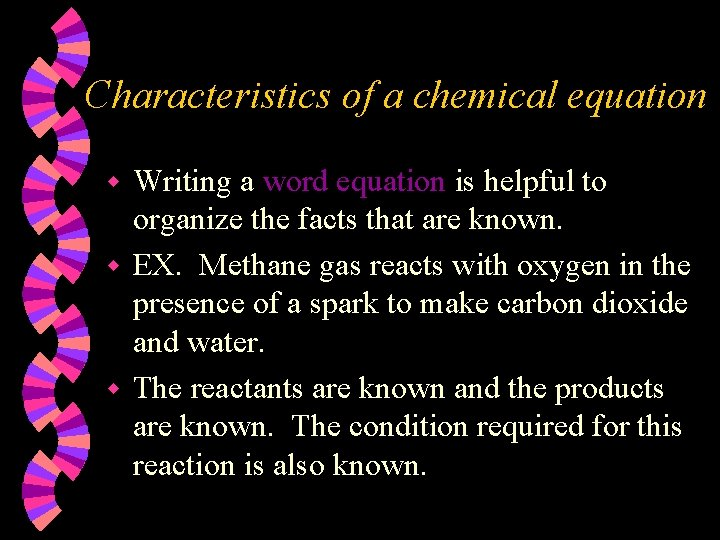Characteristics of a chemical equation Writing a word equation is helpful to organize the facts that are known. w EX. Methane gas reacts with oxygen in the presence of a spark to make carbon dioxide and water. w The reactants are known and the products are known. The condition required for this reaction is also known. w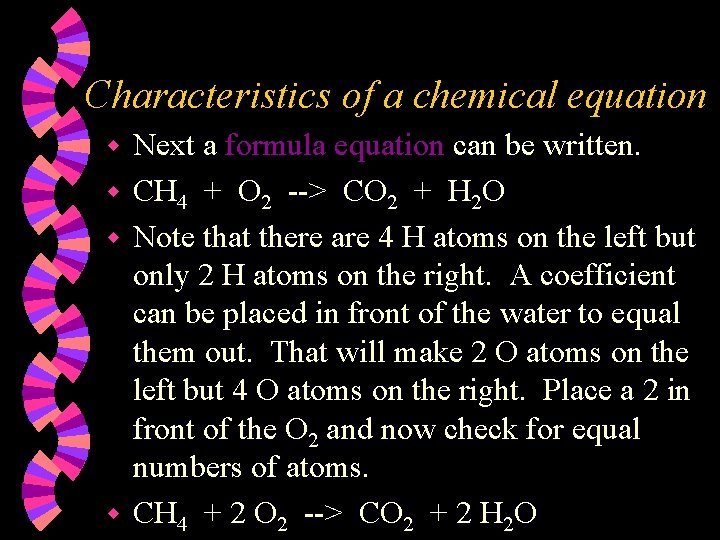Characteristics of a chemical equation Next a formula equation can be written. w CH 4 + O 2 --> CO 2 + H 2 O w Note that there are 4 H atoms on the left but only 2 H atoms on the right. A coefficient can be placed in front of the water to equal them out. That will make 2 O atoms on the left but 4 O atoms on the right. Place a 2 in front of the O 2 and now check for equal numbers of atoms. w CH 4 + 2 O 2 --> CO 2 + 2 H 2 O wSignificance of a chemical equation The coefficients indicate the relative amounts of reactants and products. The lowest whole number ratio is shown. w The relative masses can be determined from the coefficients. Once done, the law of conservation of mass can be shown to be true. wLooking at a balanced equation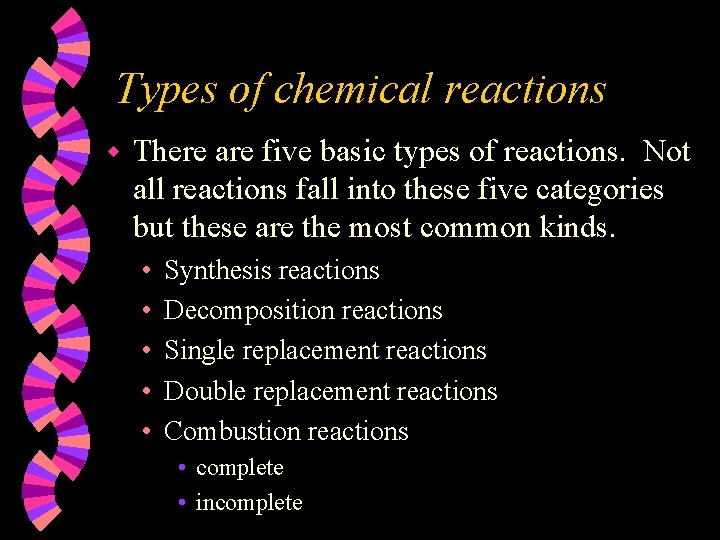Types of chemical reactions w There are five basic types of reactions. Not all reactions fall into these five categories but these are the most common kinds. • • • Synthesis reactions Decomposition reactions Single replacement reactions Double replacement reactions Combustion reactions • complete • incomplete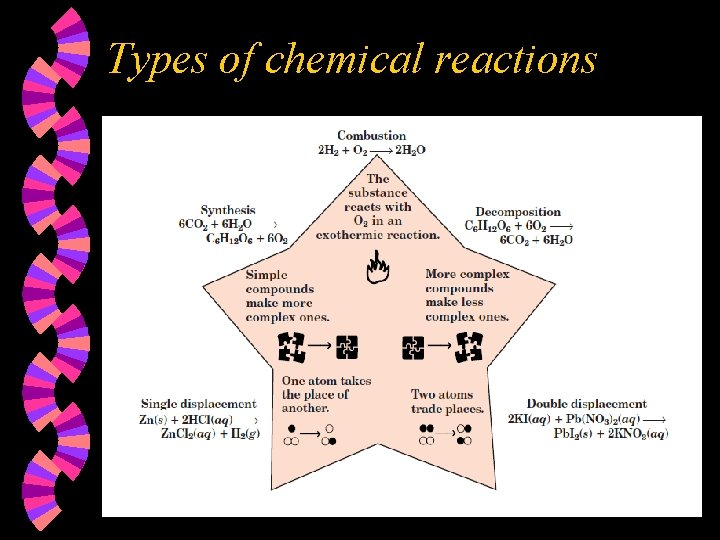Types of chemical reactionsSynthesis reactions Are also known as composition reactions or as direct combination reactions w Occur when 2 or more elements or small compounds combine to form 1 larger compound. w A + X --> AX (may or may not have subscripts) wExamples of synthesis reactions with sulfur to form sulfides • Fe + S --> Fe 2 S 3 w reactions with oxygen to form oxides • S + O 2 --> SO 2 w reactions of metals with halogens to form salts • 2 Na + Cl 2 --> 2 Na. Cl w reactions of oxides of active metals with water to form hydroxides • Ca. O + H 2 O --> Ca(OH)2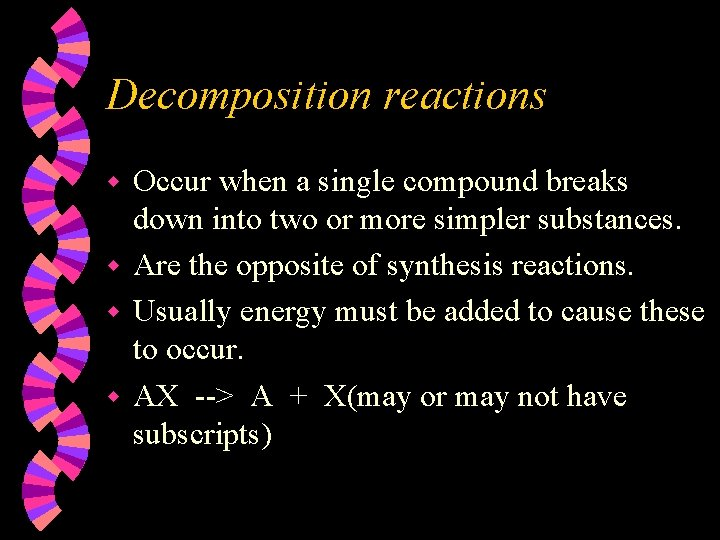Decomposition reactions Occur when a single compound breaks down into two or more simpler substances. w Are the opposite of synthesis reactions. w Usually energy must be added to cause these to occur. w AX --> A + X(may or may not have subscripts) wExamples of decomposition reactions w decomposition of binary compounds • 2 H 2 O w electricity > 2 H 2 + O 2 (electrolysis) decomposition of metal carbonates • Ca. CO 3 --> Ca. O + CO 2 w decomposition of metal hydroxides • Ca(OH)2 --> Ca. O + H 2 O w decomposition of metal chlorates • 2 KCl. O 3 --> 2 KCl + 3 O 2 w decomposition of acids • H 2 CO 3 --> H 2 O + CO 2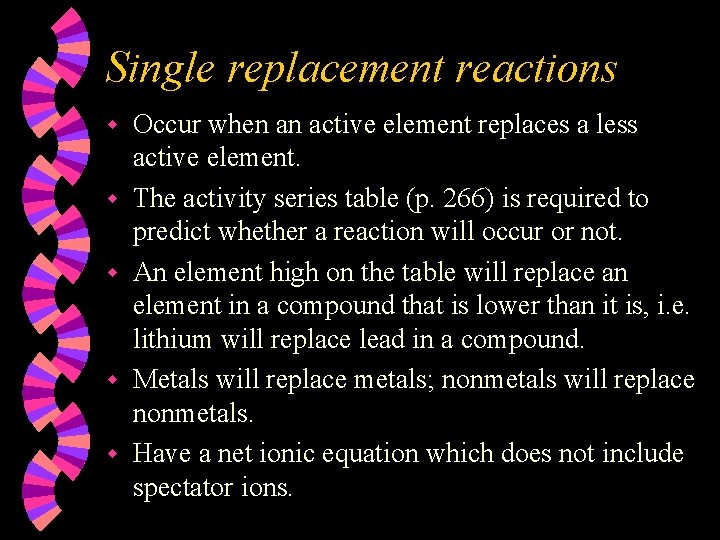Single replacement reactions w w w Occur when an active element replaces a less active element. The activity series table (p. 266) is required to predict whether a reaction will occur or not. An element high on the table will replace an element in a compound that is lower than it is, i. e. lithium will replace lead in a compound. Metals will replace metals; nonmetals will replace nonmetals. Have a net ionic equation which does not include spectator ions.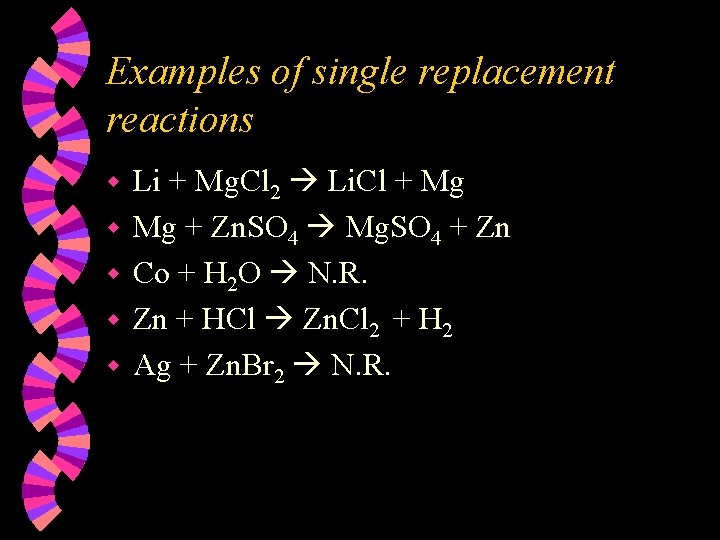Examples of single replacement reactions w w w Li + Mg. Cl 2 Li. Cl + Mg Mg + Zn. SO 4 Mg. SO 4 + Zn Co + H 2 O N. R. Zn + HCl Zn. Cl 2 + H 2 Ag + Zn. Br 2 N. R.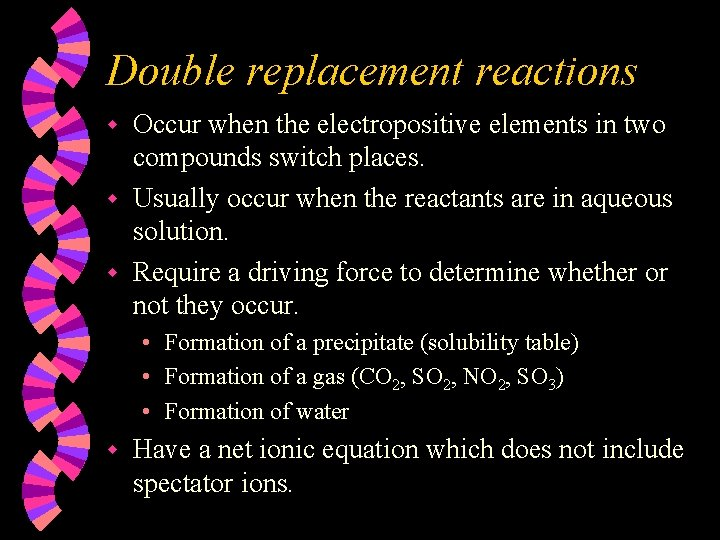Double replacement reactions Occur when the electropositive elements in two compounds switch places. w Usually occur when the reactants are in aqueous solution. w Require a driving force to determine whether or not they occur. w • Formation of a precipitate (solubility table) • Formation of a gas (CO 2, SO 2, NO 2, SO 3) • Formation of water w Have a net ionic equation which does not include spectator ions.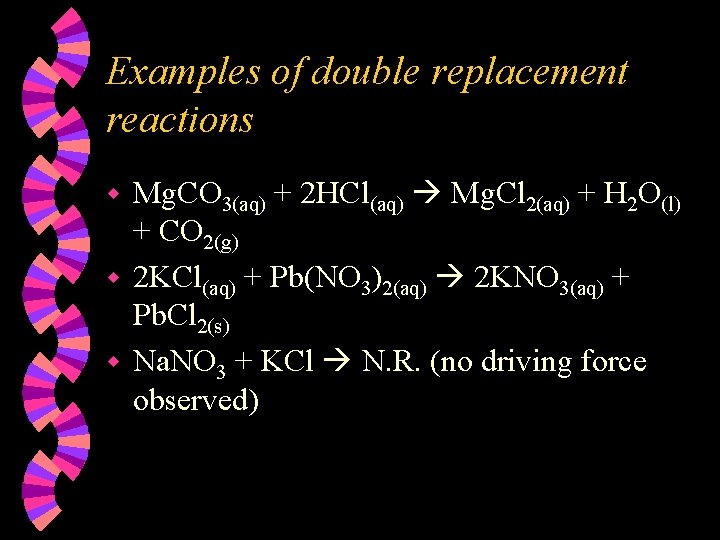Examples of double replacement reactions Mg. CO 3(aq) + 2 HCl(aq) Mg. Cl 2(aq) + H 2 O(l) + CO 2(g) w 2 KCl(aq) + Pb(NO 3)2(aq) 2 KNO 3(aq) + Pb. Cl 2(s) w Na. NO 3 + KCl N. R. (no driving force observed) w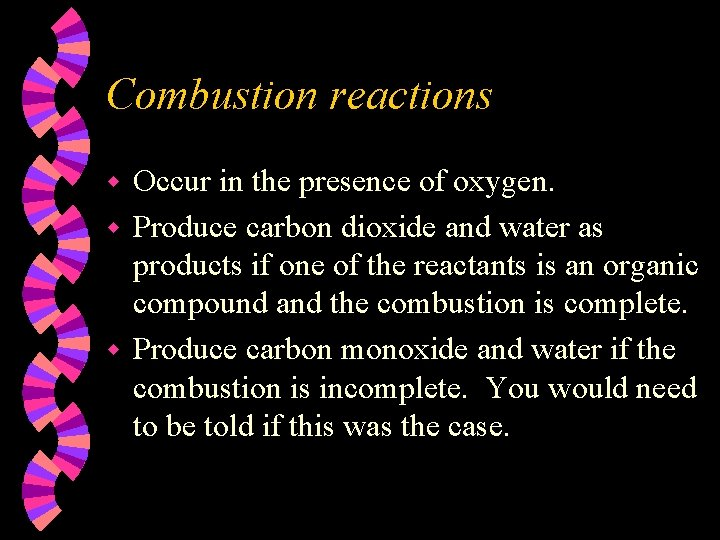Combustion reactions Occur in the presence of oxygen. w Produce carbon dioxide and water as products if one of the reactants is an organic compound and the combustion is complete. w Produce carbon monoxide and water if the combustion is incomplete. You would need to be told if this was the case. w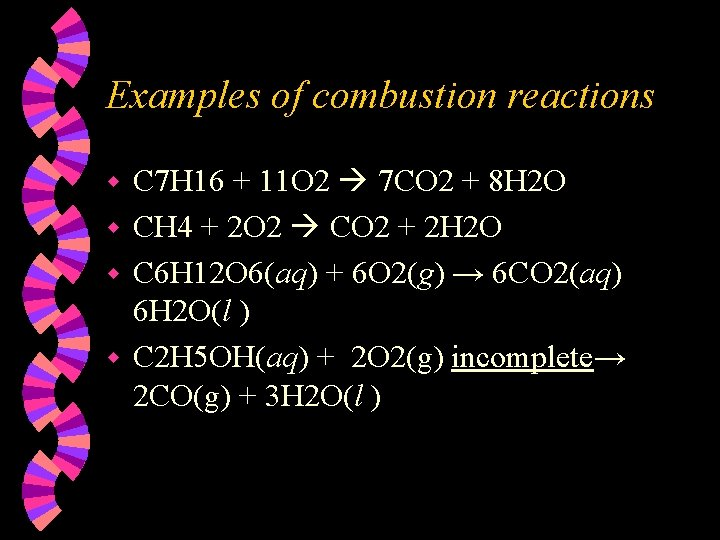Examples of combustion reactions C 7 H 16 + 11 O 2 7 CO 2 + 8 H 2 O w CH 4 + 2 O 2 CO 2 + 2 H 2 O w C 6 H 12 O 6(aq) + 6 O 2(g) → 6 CO 2(aq) 6 H 2 O(l ) w C 2 H 5 OH(aq) + 2 O 2(g) incomplete→ 2 CO(g) + 3 H 2 O(l ) wMore types of reactions Acid-base reactions are also called neutralization reactions because they produce a salt and water. w Precipitation reactions are those which produce a precipitate as a product (double replacement reactions). w Oxidation-reduction reactions are those in which two of the reacting atoms have their charges changed. One is oxidized and one is reduced. w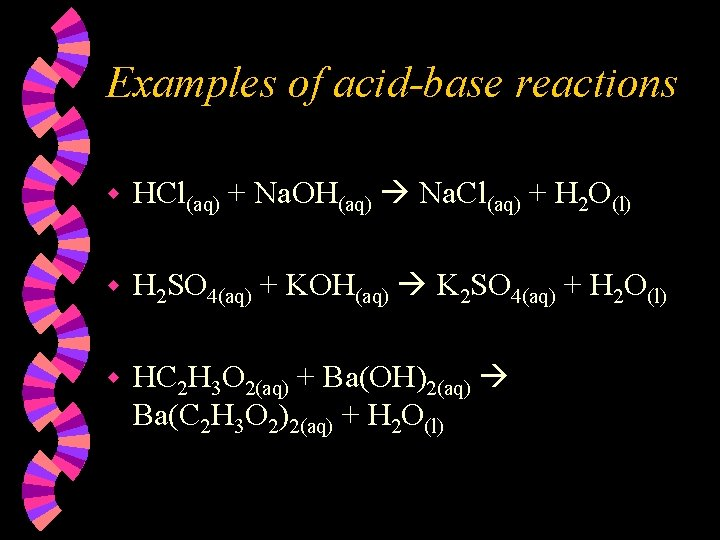Examples of acid-base reactions w HCl(aq) + Na. OH(aq) Na. Cl(aq) + H 2 O(l) w H 2 SO 4(aq) + KOH(aq) K 2 SO 4(aq) + H 2 O(l) w HC 2 H 3 O 2(aq) + Ba(OH)2(aq) Ba(C 2 H 3 O 2)2(aq) + H 2 O(l)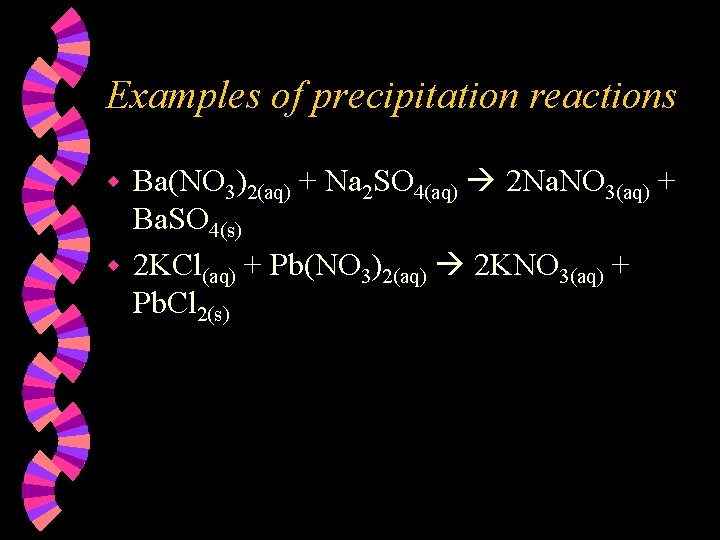Examples of precipitation reactions Ba(NO 3)2(aq) + Na 2 SO 4(aq) 2 Na. NO 3(aq) + Ba. SO 4(s) w 2 KCl(aq) + Pb(NO 3)2(aq) 2 KNO 3(aq) + Pb. Cl 2(s) w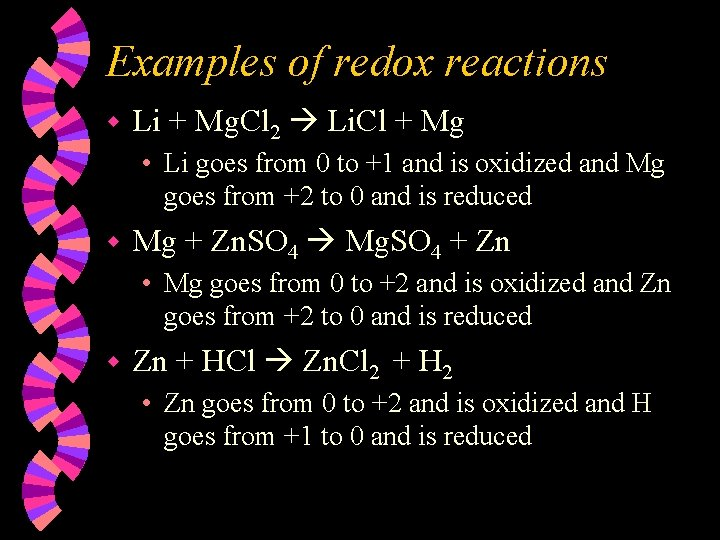Examples of redox reactions w Li + Mg. Cl 2 Li. Cl + Mg • Li goes from 0 to +1 and is oxidized and Mg goes from +2 to 0 and is reduced w Mg + Zn. SO 4 Mg. SO 4 + Zn • Mg goes from 0 to +2 and is oxidized and Zn goes from +2 to 0 and is reduced w Zn + HCl Zn. Cl 2 + H 2 • Zn goes from 0 to +2 and is oxidized and H goes from +1 to 0 and is reduced